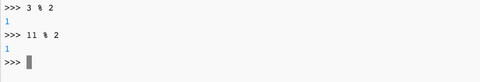Free Shipping Canada Wide over \$200
Free Shipping Canada Wide over \$200

# 3x + 1

## The simple math problem that no one can solve.

### Introduction:

• Do you know if a number is even or odd?
• Can you multiply, divide, and add?

If you answered yes to both of these questions then you have al the tools needed to understand this problem.

1. Pick any number.
2. If the number is odd, multiply the number by 3 and add 1.
3. If the number is even divide by 2.
4. Repeat.
5. Stop when you get to the number 1.

Example:

Let’s pick the number 7. We get:

7 - 22 - 11 - 34 - 17 - 52 - 26 - 13 - 40 - 20 - 10 - 5 - 16 - 8 - 4 - 2 - 1

Pick a number and see if it goes to 1. How many steps does it take?

The problem that no one can solve is whether or not this works for all numbers. What do you think?

### Coding:

It can be time consuming to find these sequences, especially as the numbers grow. Let's learn how to write some code so that the computer can do the work for us.

Lets start by answering some questions:

1. What operations do we need to perform?
2. What information do we need to know before we perform an operation?
3. When do we stop performing these operations?

#### What operations do we need to perform?

Looking at the steps in the introduction we see that in each step we need to either multiply a number by 3 and add 1 or we divide by 2. This gives us:

Operations:

• 3 * number + 1
• number / 2

#### What information do we need to know before we perform an operation?

The steps tell us that we need to know if the number is even or odd.

Information:

• even
• odd

Let's think about how the computer will know if a number is even or odd. An even number is divisible by 2 and an odd number is not. Using the console below let's find a way to do this.

Python has a remainder operator. The symbol is %. It works like this:

number % other number

will give you the remainder when "number" is divided by "other number"

Pick an even number. Say 12. If we type 12 % 2. It will give us the remainder when 12 is divided by 2. Since 12 is even we should get 0. You can test it below in the python console.  Here are some examples:You can also try some odd numbers. They will give the answer 1. Here are some examples:This gives us the following:

Information

• even: number % 2 = 0
• odd: number % 2 = 1

#### When do we stop performing these operations?

Our steps say that we stop when we reach the number 1. We do this because if we continued on we would be in a loop forever:

• 4: even (divide by 2)
• 2: even (divide by 2)
• 1: odd (multiply by 3 and add 1)
• 4: even (divide by 2)
• 2: even (divide by 2)
• ...

4 - 2 - 1 - 4 - 2 - 1 - 4 - 2 - 1 - 4 - 2 - 1 - 4 - 2 - 1

#### Summary

1. What operations do we need to perform?
• 3 * number + 1
• number / 2
• What information do we need to know before we perform an operation?
• even: number % 2 = 0
• odd: number % 2 = 1
• When do we stop performing these operations?
• stop when number = 1